## How to Measure Consistency?

What is the meaning that our opinion is consistent? How do we measure the consistency of subjective judgment? At the end of this section will be able to answer those questions.

Let us look again on John's judgment that we discussed in the previous section . Is John judgment consistent or not?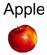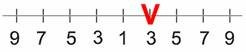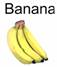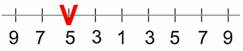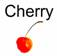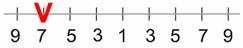First he prefers Banana to Apple. Thus we say that for John, Banana has greater value than Apple. We write it as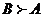.

Next, he prefers Apple to Cherry. For him, Apple has greater value than Cherry. We write it as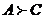.

Sinceand, logically, we hope that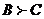or Banana must be preferable than Cherry. This logic of preference is called transitive property. If John answers in the last comparison is transitive (that he like Banana more than Cherry), then his judgment is consistent. On the contrary, if John prefers Cherry to Banana then his answer is inconsistent. Thus consistency is closely related to the transitive property.

A comparison matrix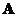is said to be consistent if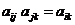for all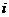,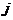and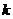. However, we shall not force the consistency. For example,has value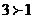andhas value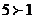, we shall not insist thatmust have value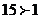. This too much consistency is undesirable because we are dealing with human judgment. To be called consistent , the rank can be transitive but the values of judgment are not necessarily forced to multiplication formula.

Prof. Saaty proved that for consistent reciprocal matrix, the largest Eigen value is equal to the size of comparison matrix, or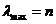. Then he gave a measure of consistency, called Consistency Index as deviation or degree of consistency using the following formula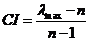Thus, in our previous example, we have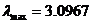and the size of comparison matrix is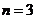, thus the consistency index is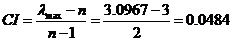Knowing the Consistency Index, the next question is how do we use this index? Again, Prof. Saaty proposed that we use this index by comparing it with the appropriate one. The appropriate Consistency index is called Random Consistency Index (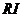).

He randomly generated reciprocal matrix using scale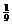,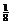, ...,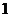, ...,8, 9 (similar to the idea of Bootstrap) and get the random consistency index to see if it is about 10% or less. The average random consistency index of sample size 500 matrices is shown in the table below

Table 8: Random Consistency Index ()

 n 1 2 3 4 5 6 7 8 9 10 RI 0 0 0.58 0.9 1.12 1.24 1.32 1.41 1.45 1.49

Then, he proposed what is called Consistency Ratio, which is a comparison between Consistency Index and Random Consistency Index, or in formula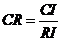If the value of Consistency Ratio is smaller or equal to 10%, the inconsistency is acceptable. If the Consistency Ratio is greater than 10%, we need to revise the subjective judgment.

For our previous example, we have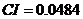andforis 0.58, then we have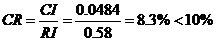. Thus, John's subjective evaluation about his fruit preference is consistent.

So far, in AHP we are only dealing with paired comparison of criteria or alternative but not both. In next section, I show an example to use both criteria and alternative in two levels of AHP.